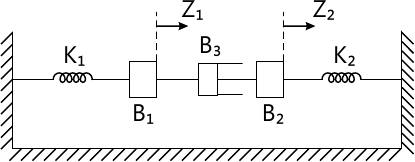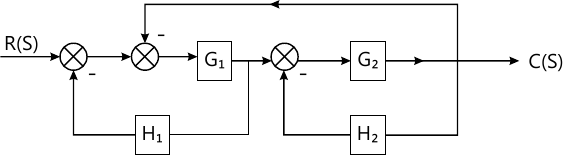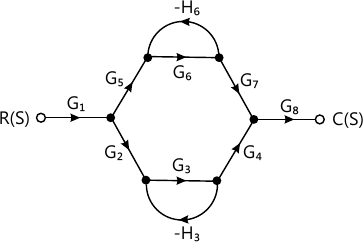Total marks: --
Total time: --
INSTRUCTIONS
(1) Assume appropriate data and state your reasons
(2) Marks are given to the right of every question
(3) Draw neat diagrams wherever necessary

1 (a) Distinguish between open-loop and closed loop systems with examples.
5 M
1 (b) Explain the requirements of a control system.
5 M
1 (c) Explain following controller. State its characteristics:
i) Proportional plus derivative control action
ii) Proportional plus integral plus derivative control action.
10 M

2 (a) Write the equilibrium equations for the mechanical system shown in Fig Q2(a), hence obtain the F-I analogous system.10 M
2 (b) Obtain the transfer function of field controlled DC motor.
10 M

3 (a) Reduce the block diagram and obtain its transfer function $\dfrac {C(s)}{R(s)}$10 M
3 (b) Find $\dfrac {C(s)} {R(s)}$ by Mason's gain formula.10 M

4 (a) Obtain an expression for time response of the first order system subjected to unit step input.
8 M
4 (b) Determine the damping ratio and natural frequency for the system whose maximum overshoot response is 0.2 and peak time is 1 sec. Find rise time and settling time.
6 M
4 (c) State whether the system is stable or unstable s6+2s5+8s4+123+20s2+16s+16=0 using Routh's stability criterion.
6 M

5 (a) Sketch the polar plot of TF $G(s)H(s)= \dfrac {1}{(1+5s)(1+10s)}$
6 M
5 (b) Sketch the Nyquist plot for a system, whose transfer function, $G(s) H(s)= \dfrac {K}{s(s+4)(s+8)}$ Determine the range of values of K for which the system in stable.
14 M

6 For a system $G(s)H(s)= \dfrac {242(s+5)} {s(s+1)(s^2+5s+121)}$ sketch the Bode plot. Find ωpc and ωgc GM, PM. Comment on stability.
20 M

7 For a unity feedback system, $G(s) H(s)= \dfrac {K}{s(s+4)(s+2)}$ sketch the rough nature of the root locus, showing all details on it.
20 M

8 (a) What is compensation? How are compensators classified?
6 M
8 (b) Write notes on:
8 (c) A system is governed by the differential equation $\dfrac {d^3y}{dt^3}+ 6 \dfrac {d^2y}{dt^2}+ 11 \dfrac {dy}{dt}+10 =8u(t)$ where y is the output and u is the input of the system. Obtain a state space representation of the system.